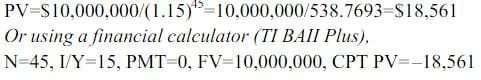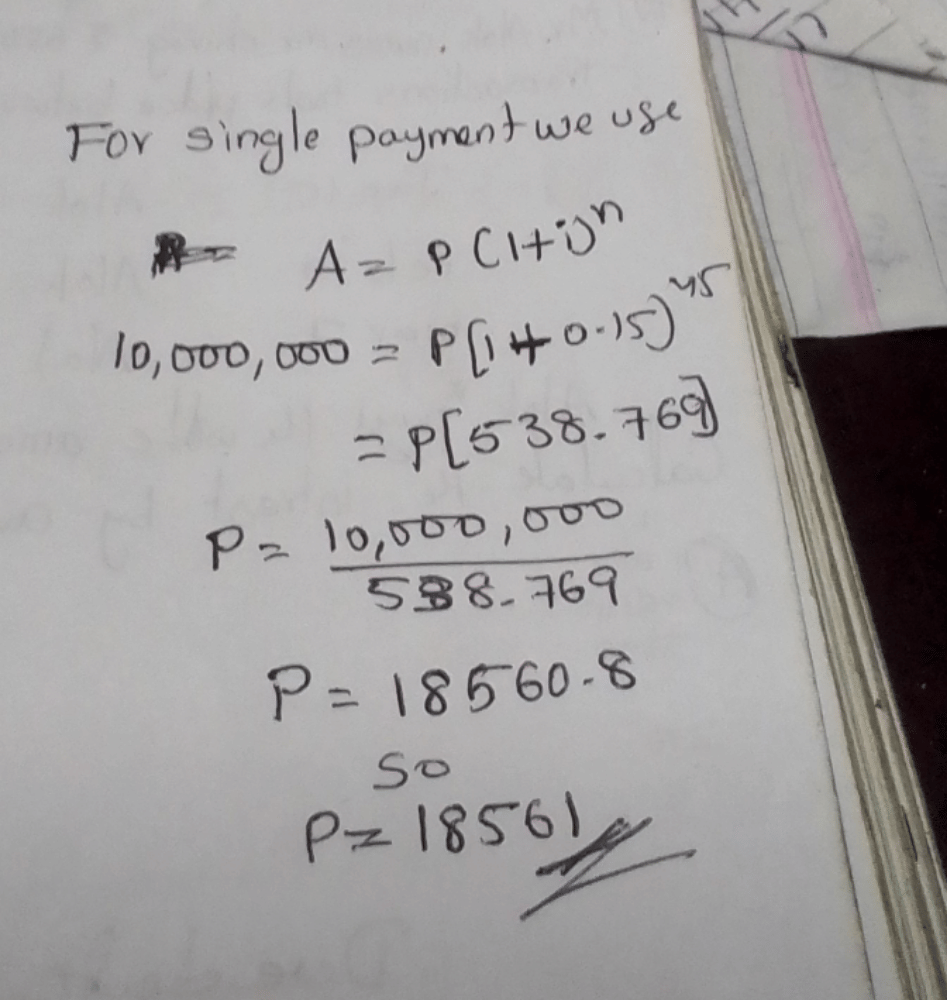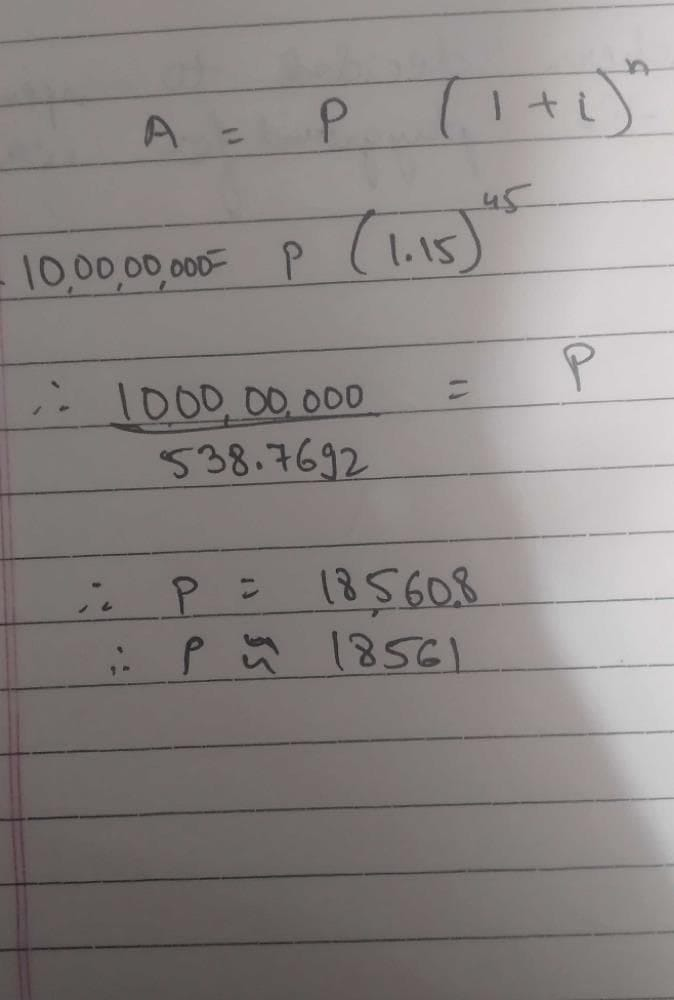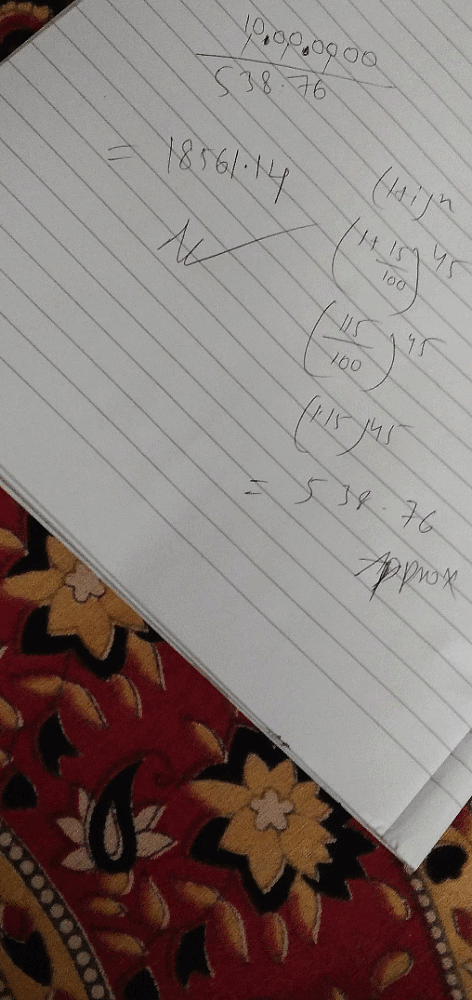Suppose an investor wants to have \$10 million...

### Related TestSuppose an investor wants to have \$10 million to retire 45 years from now. How much would she have to invest today with an annual rate of return equal to 15 percent?
• a)
\$18,561
• b)
\$17,844
• c)
\$20,003
• d)
\$21,345Patel Jaydatt1 Crore+ students have signed up on EduRev. Have you?Shaik Sadiq
Suppose an investor wants to have \$10 million to retire 45 years from now. How much would she have to invest today with an annual rate of return equal to 15 percent?a)\$18,561b)\$17,844c)\$20,003d)\$21,345Correct answer is option 'A'. Can you explain this answer?View courses related to this question Explore CA Foundation courses
 Explore CA Foundation coursesView courses related to this questionKashish KatyarVikas Vicky
Suppose an investor wants to have \$10 million to retire 45 years from now. How much would she have to invest today with an annual rate of return equal to 15 percent?a)\$18,561b)\$17,844c)\$20,003d)\$21,345Correct answer is option 'A'. Can you explain this answer?Nandani
BMonu Gautam
Suppose an investor wants to have \$10 million to retire 45 years from now. How much would she have to invest today with an annual rate of return equal to 15 percent?a)\$18,561b)\$17,844c)\$20,003d)\$21,345Correct answer is option 'A'. Can you explain this answer?
A= P( 1+i)^n
1 million=10,00,000
10 million=1,00,00,000
1,00,00,000 = P (1.15)^45
1,00,00,000) = P (538.769268949)
1,00,00,000/538.7692 =P
18560.82 =PTanushree Dubey
Using formula for Compound interestA=P(1 i)^nHere,A=10,000,000 i=0.15 n=45 10,000,000=P(1.15)^45 P = 18,560.82 ~ 18,561

Question Description
Suppose an investor wants to have \$10 million to retire 45 years from now. How much would she have to invest today with an annual rate of return equal to 15 percent?a)\$18,561b)\$17,844c)\$20,003d)\$21,345Correct answer is option 'A'. Can you explain this answer? for CA Foundation 2023 is part of CA Foundation preparation. The Question and answers have been prepared according to the CA Foundation exam syllabus. Information about Suppose an investor wants to have \$10 million to retire 45 years from now. How much would she have to invest today with an annual rate of return equal to 15 percent?a)\$18,561b)\$17,844c)\$20,003d)\$21,345Correct answer is option 'A'. Can you explain this answer? covers all topics & solutions for CA Foundation 2023 Exam. Find important definitions, questions, meanings, examples, exercises and tests below for Suppose an investor wants to have \$10 million to retire 45 years from now. How much would she have to invest today with an annual rate of return equal to 15 percent?a)\$18,561b)\$17,844c)\$20,003d)\$21,345Correct answer is option 'A'. Can you explain this answer?.
Solutions for Suppose an investor wants to have \$10 million to retire 45 years from now. How much would she have to invest today with an annual rate of return equal to 15 percent?a)\$18,561b)\$17,844c)\$20,003d)\$21,345Correct answer is option 'A'. Can you explain this answer? in English & in Hindi are available as part of our courses for CA Foundation. Download more important topics, notes, lectures and mock test series for CA Foundation Exam by signing up for free.
Here you can find the meaning of Suppose an investor wants to have \$10 million to retire 45 years from now. How much would she have to invest today with an annual rate of return equal to 15 percent?a)\$18,561b)\$17,844c)\$20,003d)\$21,345Correct answer is option 'A'. Can you explain this answer? defined & explained in the simplest way possible. Besides giving the explanation of Suppose an investor wants to have \$10 million to retire 45 years from now. How much would she have to invest today with an annual rate of return equal to 15 percent?a)\$18,561b)\$17,844c)\$20,003d)\$21,345Correct answer is option 'A'. Can you explain this answer?, a detailed solution for Suppose an investor wants to have \$10 million to retire 45 years from now. How much would she have to invest today with an annual rate of return equal to 15 percent?a)\$18,561b)\$17,844c)\$20,003d)\$21,345Correct answer is option 'A'. Can you explain this answer? has been provided alongside types of Suppose an investor wants to have \$10 million to retire 45 years from now. How much would she have to invest today with an annual rate of return equal to 15 percent?a)\$18,561b)\$17,844c)\$20,003d)\$21,345Correct answer is option 'A'. Can you explain this answer? theory, EduRev gives you an ample number of questions to practice Suppose an investor wants to have \$10 million to retire 45 years from now. How much would she have to invest today with an annual rate of return equal to 15 percent?a)\$18,561b)\$17,844c)\$20,003d)\$21,345Correct answer is option 'A'. Can you explain this answer? tests, examples and also practice CA Foundation tests.

• ### A certain sum of money was invested at simple rate of interest for three ye... more(Scan QR code)